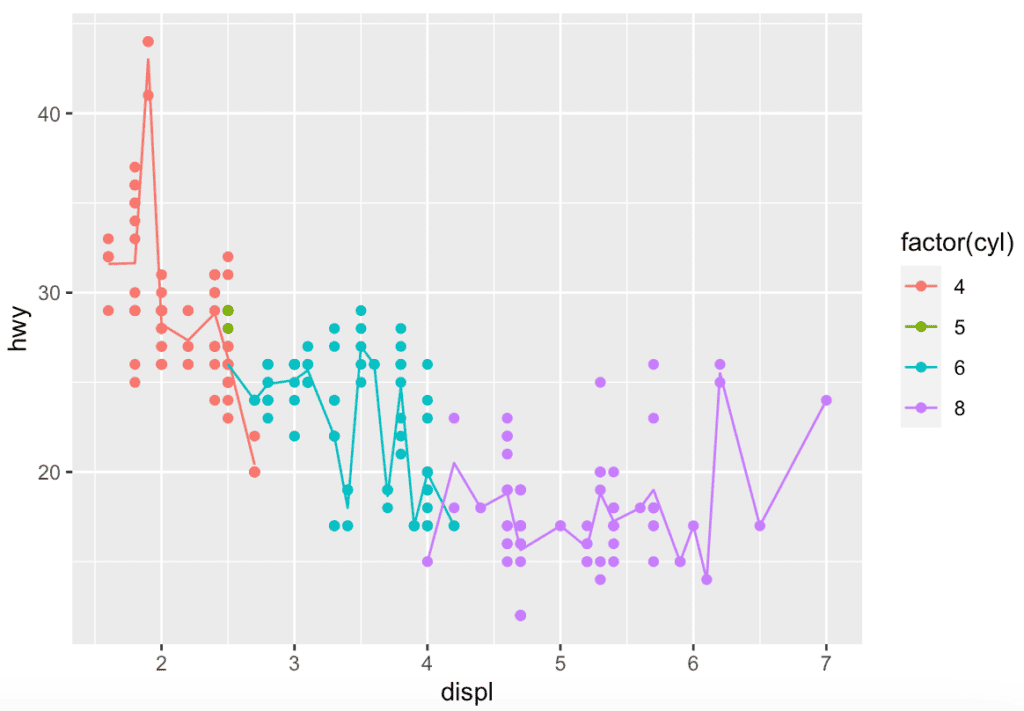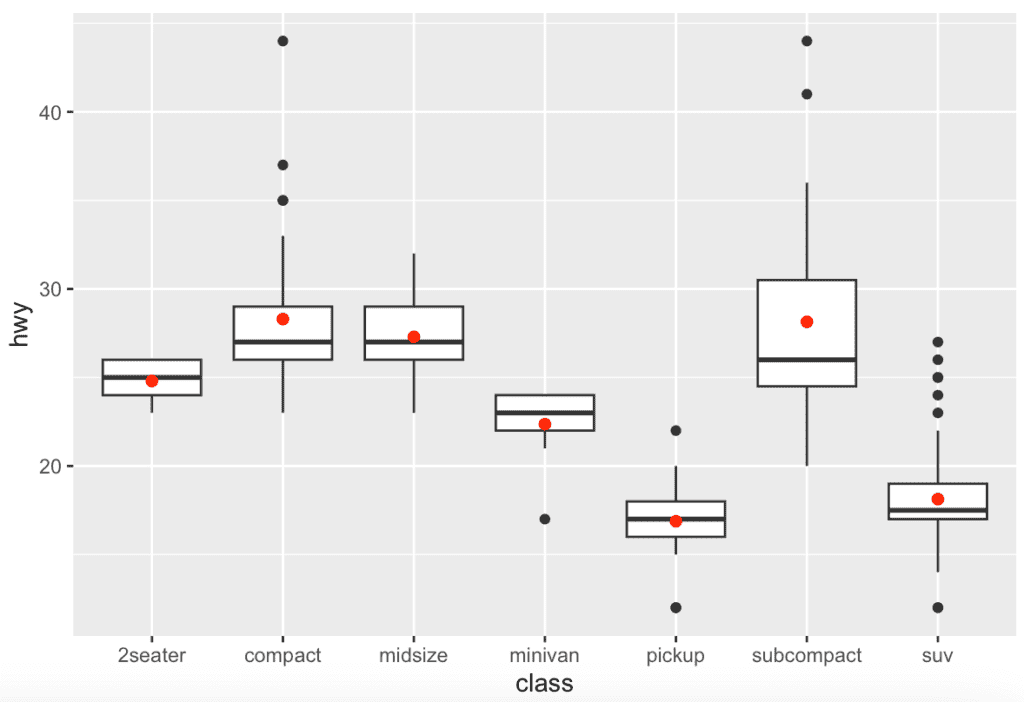# How to Use the stat_summary() Function in R

The stat_summary() function in R “allows for tremendous flexibility in the specification of summary functions”.

The stat_summary() function calculates various summary statistics for data points, such as the mean, median, maximum, minimum, or standard deviation. It takes a summary function as an argument, such as mean, median, max, min, sd, q1, or q3, to name a few.

## Syntax

``````stat_summary(mapping = NULL, data = NULL,
geom = "pointrange", position = "identity")``````

## Parameters

1. mapping: Aesthetic mapping, usually constructed with aes or aes_string.
2. data: A layer-specific dataset – is only needed if you want to override the plot defaults.
3. geom: The geometric object to use to display the data.
4. position: The position adjustment to use for overlapping points on this layer.

## Example 1: R program of stat_summary() function

The stat_summary() function is used in combination with the geom_point() or geom_line() functions to add a summary point or line to a graph. It is useful for quickly visualizing summary statistics across different groups or categories in the data.

``````library(ggplot2)

ggplot(mpg, aes(x = displ, y = hwy, color = factor(cyl))) +
geom_point() +
stat_summary(fun = mean, geom = "line", aes(group = cyl))``````

Output## Example 2: The stat_summary() function from ggplot()

``````library(ggplot2)

ggplot(mpg, aes(class, hwy)) +
geom_boxplot() +
stat_summary(fun = "mean", geom = "point", shape = 20, size = 3, color = "red")``````

OutputThat’s it.